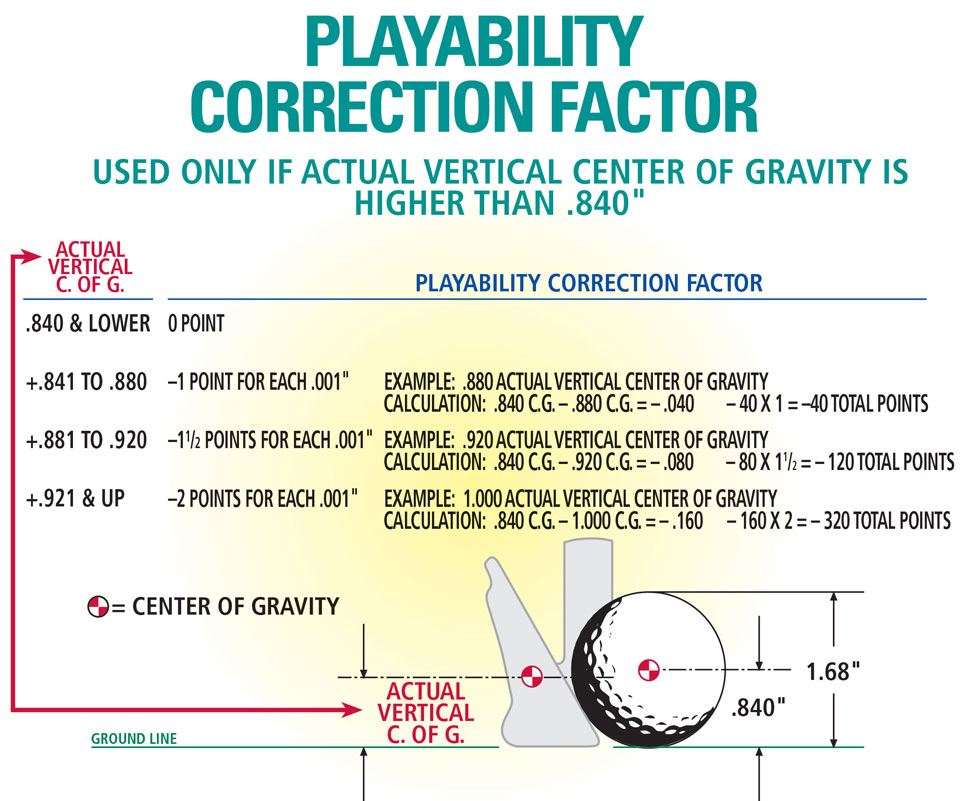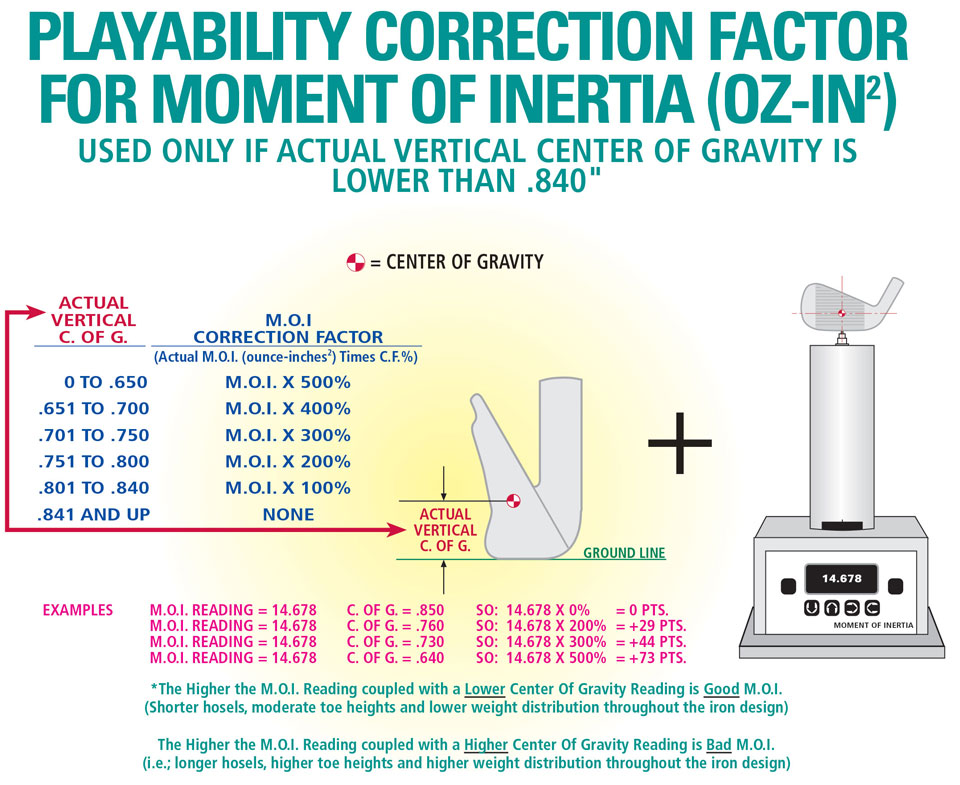# Method of Determining MPF

Calculating the MPF is a four part process. In this article we will go over each step thoroughly and explain it in greater detail.

### Steps To Determining MPF

#### Part 1

Subtract the Actual Vertical Center of Gravity from the “C” Dimension

#### Part 2

Subtract any Center of Gravity Correction Factor Points

#### Part 3

Add in the Playability Correction Factor Points for Moment of Inertia

#### Part 4

Add 30 Points to MPF for Every Iron (Measuring Correction Factor)

This is not a complicated formula or method to determine MPF. However, a number of measurements and calculations are required to get the final Playability number. The rest of the discussion here will explain exactly how to accomplish this.

### Actual Vertical Center of Gravity

When a golf club is balanced on its face as shown below, The center of gravity location found is rarely, if ever, the Actual Center of Gravity. What we are measuring is the Basic Vertical Center of Gravity and all we know from this is that the Actual Center of Gravity is located somewhere on the same plane.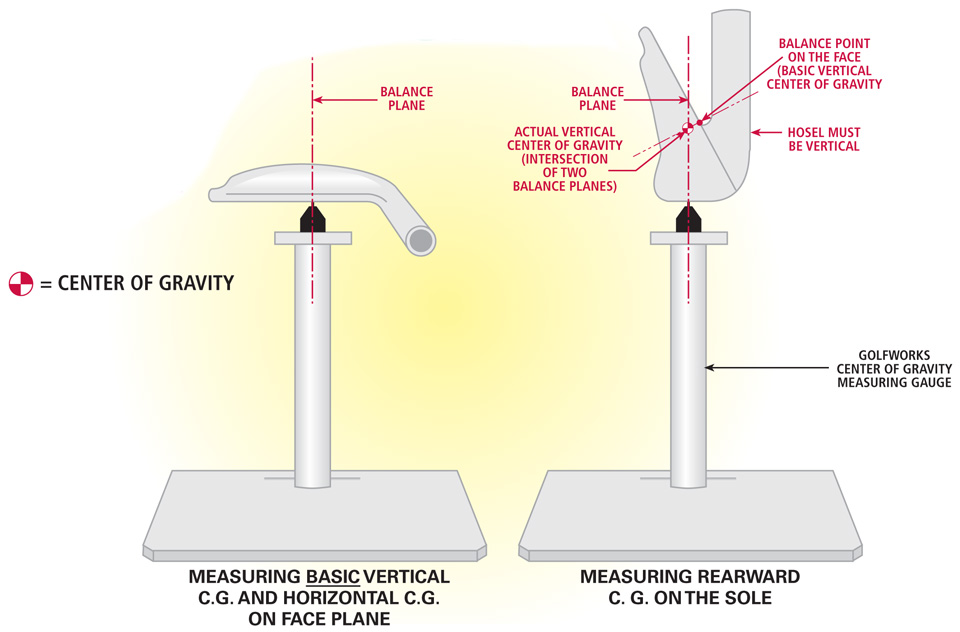Note: The Center of Gravity is located where the two balance planes intersect. The intersection point (actual c.g. location) can either be inside or outside the head mass.What the same plane means is that if we extended a 90° line through the balance point on the face it would intersect the Actual Center of Gravity. The problem is that we still do not have enough information to find out exactly where the intersection would be.

The additional piece of information that we need can be found by locating the Rearward Center of Gravity. Looking at the figure above which shows a Rearward Center of Gravity balancing point.

### Defining Rearward Center of Gravity

Now look below to see a definition of Rearward Center of Gravity. We now have two marks on the clubhead. One mark on the face (Basic Vertical Center of Gravity) and one mark on the sole (Rearward Center of Gravity).Note: The Rearward Center of Gravity is measured in this manner so that it is a relatively simple trigonometry function to find a clubhead’s Actual Vertical Center of Gravity. When measuring the Rearward Center of Gravity to be used as a separate and additional performance characteristic; it is measured from the Centerline of the Hosel to the Rearward Center of Gravity.A number of methods can be used to measure the actual locations of these two marks. We will look at one measuring method here to help in understanding exactly what we are measuring and where is it measured from. Next, study the diagram below, which shows how to measure both locations using a special gauge developed to measure from the correct location (where the ground line intersects the face plane).

### How To Measure Rearward And Basic Vertical Center of Gravity Location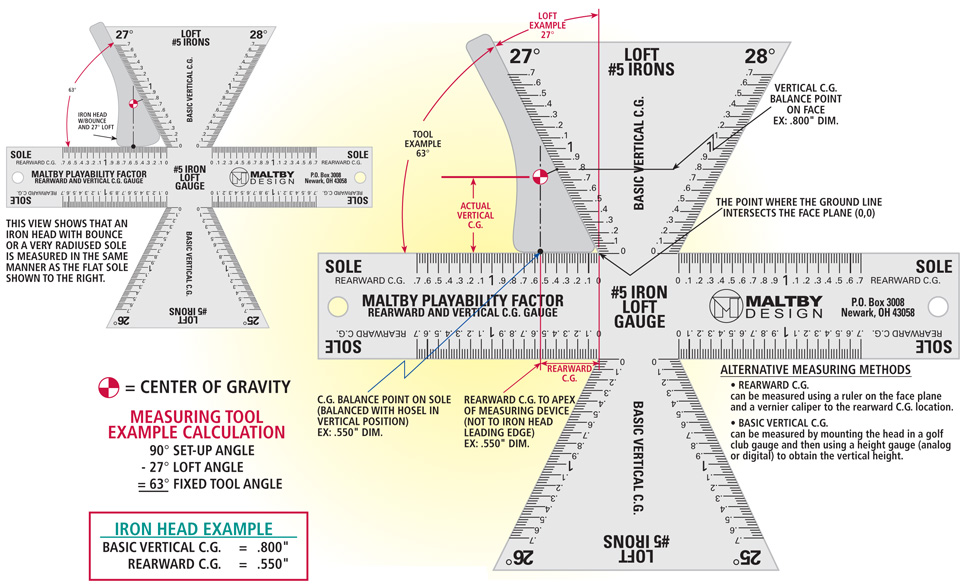This diagram shows the Actual Vertical Center of Gravity, but at this time we do not know its exact calculated dimension. We can visualize its location in the drawing because the 90° line from the balance point on the face intersects with the 90° line from the sole balance point and this intersection point is obviously the Actual Vertical Center of Gravity location.

### How To Calculate The Actual Vertical Center of Gravity Location of Irons

Below is a diagram on how to calculate the Actual Vertical Center of Gravity location of irons. Spend some time looking over this drawing to gain a solid understanding of why we need to calculate the Actual Vertical C.G. through simple trigonometry functions.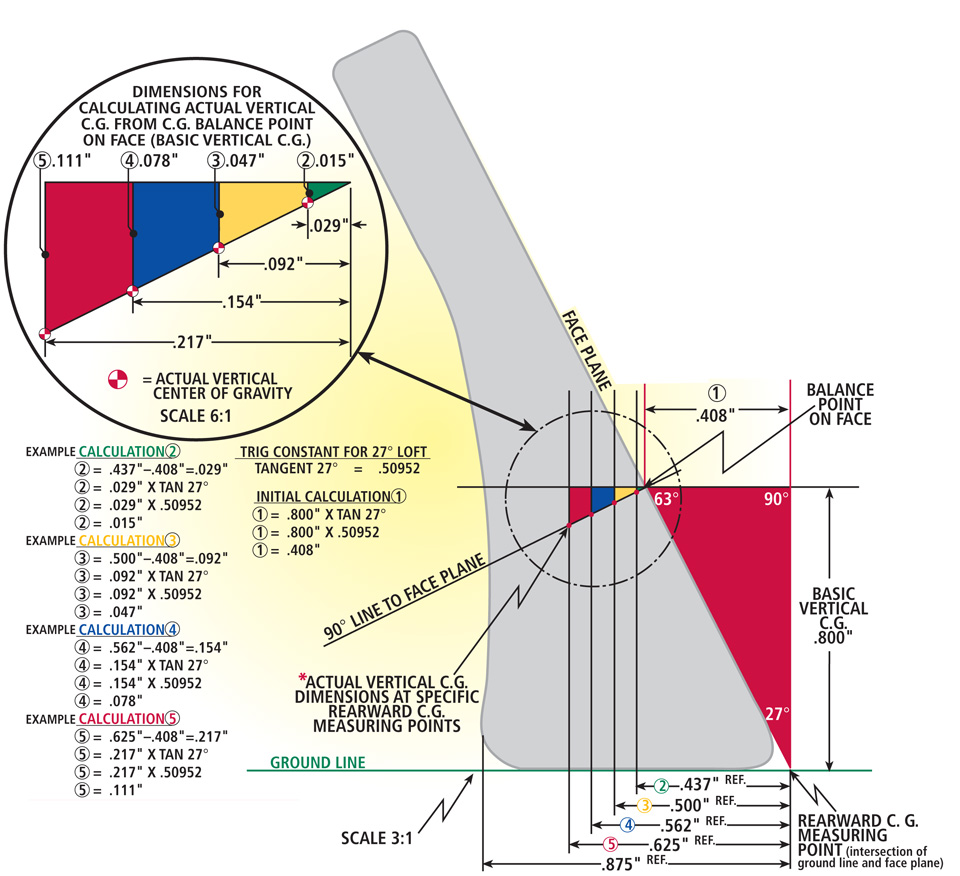Note: This drawing illustrates an example iron with a .800 basic vertical c.g. and 4 different rearward c.g. location calculations for reference.In most modern irons, the Actual Vertical C.G. height will be less than the Basic Vertical C.G. height. However, if one is calculating the Actual Vertical C.G. of some short irons (usually #8 or #9 and wedges), a number of times the Actual Vertical C.G. will be higher than the Basic Vertical C.G. because the C.G. is located outside the clubhead in front of the face.

### How To Calculate Actual Vertical Center of Gravity Location

Below shows the formula to calculate the Actual Vertical Center of Gravity location. These diagrams assigns letter characters to the specific dimensions that are required to do the calculations.

#### Key

• B – Basic Vertical Center of Gravity
• T – Tangent of #5 Iron Loft
• L – Leading Edge Measurement Point To Actual Balance Point On Face Plane
• R – Rearward Center of Gravity
• H – Horizontal Distance Of Center Of Gravity In Head Measured From Basic Vertical Center of Gravity Point On Face Plane
• D – Vertical Center Of Gravity Location Adjustment
• V – Actual Vertical Center Of Gravity

#### Step 1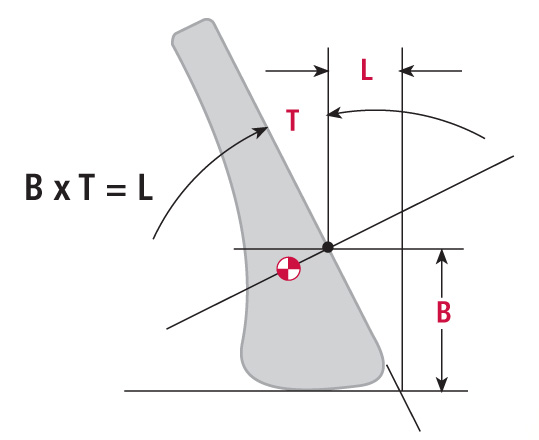### B x T = L

B – Basic Vertical Center of Gravity

T – Tangent of #5 Iron Loft

L – Leading Edge Measurement Point To Actual Balance Point On Face Plane)

#### Step 2### R – L = H

R – Rearward Center of Gravity

L – Leading Edge Measurement Point To Actual Balance Point On Face Plane

H – Horizontal Distance Of Center Of Gravity In Head Measured From Basic Vertical Center of Gravity Point On Face Plane

#### Step 3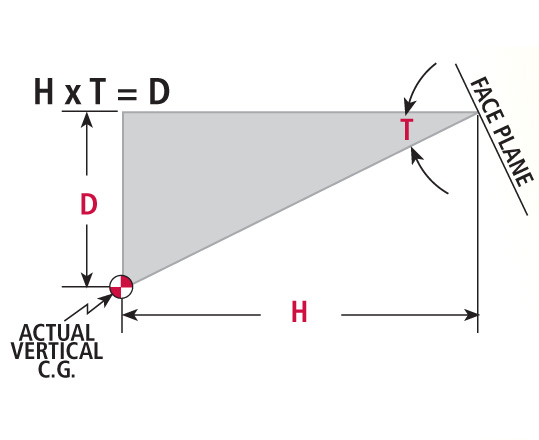### H x T = D

H – Horizontal Distance Of Center Of Gravity In Head Measured From Basic Vertical Center of Gravity Point On Face Plane

T – Tangent of #5 Iron Loft

D – Vertical Center Of Gravity Location Adjustment

#### Step 4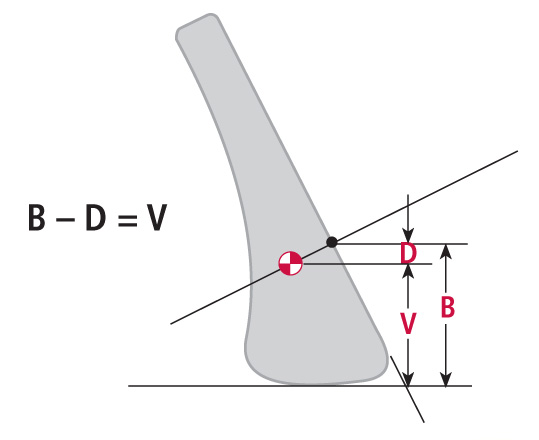### B x D = V

B – Basic Vertical Center of Gravity

D – Vertical Center Of Gravity Location Adjustment

V – Actual Vertical Center Of Gravity

### Example

#### Step 1 (B x T = L)

.800″ x TAN 27° (.50952) = .408″

#### Step 2 (R – L = H)

.562″ – .408″ = .154″

#### Step 3 (H x T = D)

.154″ x TAN 27° (.50952) = .078″

#### Step 4 (B – D = V)

.800″ – .078″ = .722″ (Actual Vertical Center of Gravity)

Go ahead and pick a loft, a Rearward and Basic Vertical Center Of Gravity out of the air and do the calculation for practice. Remember, the goal here is for anyone to pick any head and be able to accurately find the Actual Center Of Gravity location, if desired. Of course, if you want to calculate MPF, use a #5 or #6 iron.